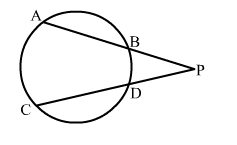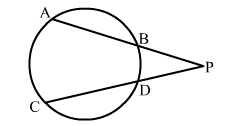# Two chords AB and CD of a circle intersect each other at P outside the circle.

Question:

Two chords AB and CD of a circle intersect each other at P outside the circle. If AB = 6 cm, BP = 2 cm and PD = 25 cm, find CD.Solution:AB and CD are two chords of a circle which intersect each other at P outside the circle.
AB = 6 cm, BP = 2 cm and PD = 2.5 cm
∴  AP × BP = CP × DP
⇒ 8 × 2 = (CD + 2.5) × 2.5  [∵ CP = CD + DP]
Let CD x cm
Thus, 8 × 2 = (CD + 2.5) × 2.5
⇒ 16 = 2.5x + 6.25
⇒ 2.5x = (16 - 6.25) = 9.75

$\Rightarrow x=\frac{9.75}{2.5}=3.9$

Hence, CD = 3.9 cm